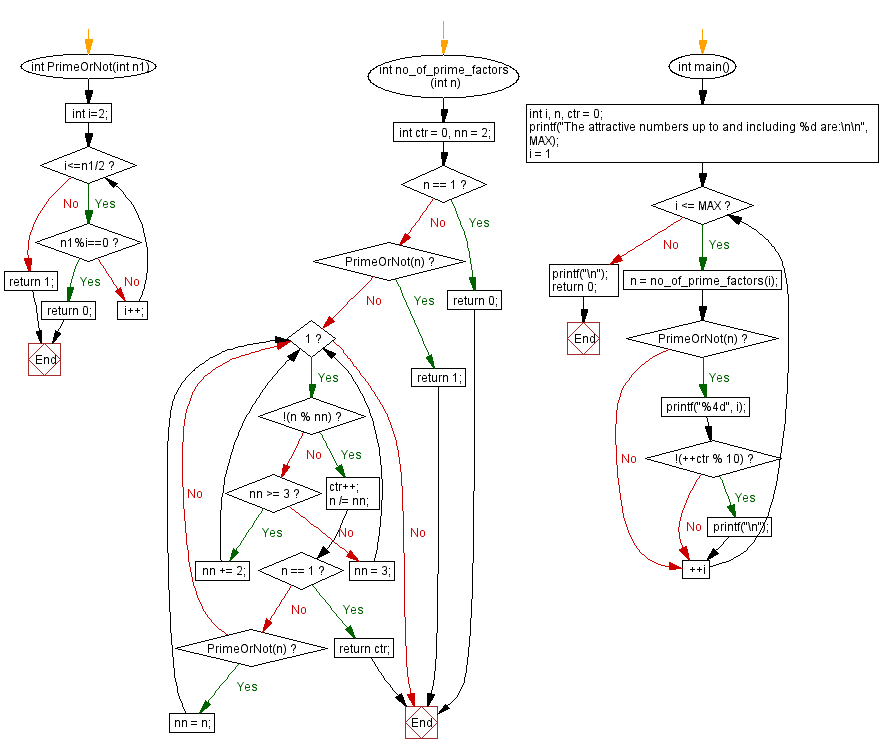﻿ C Program - Attractive numbers up to 100# C Exercises: Attractive numbers up to 100

## C Programming Challenges: Exercise-33 with Solution

A number is called an attractive number if the number of its prime factors (whether distinct or not) is also a prime number.
Example:
The number 30, whose prime decomposition is 2 × 3 × 5, is an attractive number because the number of its prime factors (3) is also a prime number.
The number 100, whose prime decomposition is 2 × 2 x 5 × 5, is not an attractive number because the number of its prime factors (4) is not a prime number.
Write a C program to generate the attractive numbers up to 100.

C Code:

``````#include <stdio.h>
#define MAX 100

int PrimeOrNot(int n1)
{
int i=2;
while(i<=n1/2)
{
if(n1%i==0)
return 0;
else
i++;
}
return 1;
}

int no_of_prime_factors(int n) {
int ctr = 0, nn = 2;
if (n == 1) return 0;
if (PrimeOrNot(n)) return 1;
while (1) {
if (!(n % nn)) {
ctr++;
n /= nn;
if (n == 1) return ctr;
if (PrimeOrNot(n)) nn = n;
}
else if (nn >= 3) nn += 2;
else nn = 3;
}
}

int main() {
int i, n, ctr = 0;
printf("The attractive numbers up to and including %d are:\n\n", MAX);
for (i = 1; i <= MAX; ++i) {
n = no_of_prime_factors(i);
if (PrimeOrNot(n)) {
printf("%4d", i);
if (!(++ctr % 10)) printf("\n");
}
}
printf("\n");
return 0;
}
``````

Sample Output:

```The attractive numbers up to and including 100 are:

1   2   3   4   5   6   7   8   9  10
11  12  13  14  15  17  18  19  20  21
22  23  25  26  27  28  29  30  31  32
33  34  35  37  38  39  41  42  43  44
45  46  47  48  49  50  51  52  53  55
57  58  59  61  62  63  65  66  67  68
69  70  71  72  73  74  75  76  77  78
79  80  82  83  85  86  87  89  91  92
93  94  95  97  98  99
```

Flowchart:## C Programming Code Editor:

Contribute your code and comments through Disqus.

Previous C Programming Exercise: Prime number in strictly descending decimal digit order.
Next C Programming Exercise: Colorful numbers.

What is the difficulty level of this exercise?

Test your Programming skills with w3resource's quiz.

﻿